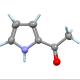## A probabilty puzzle

Suppose that we have surveyed a certain population of people, and have determined the following probabilities:

• The probability that a person likes anchovies is$a$.
• The probability that a person likes to read books is$b$.
• The probability that a person likes carpets is$c$.

Suppose we also know that these probabilities are all independent, that is, they do not influence each other at all. Wether a person likes anchovies has nothing to do with whether they like to read or whether they like carpets, and so on. When probabilities are independent, it makes sense to multiply them: for example, the probability that someone likes both anchovies and carpets is the product$ac$.

• What is the probability that a randomly chosen person likes neither anchovies nor books?

• What is the probability that a randomly chosen person likes none of these three things?

• Can you generalize? What if we had four, five, or more independent probabilities for things people might like; what would be the probability that a random person didn’t like any of the things?Associate Professor of Computer Science at Hendrix College. Functional programmer, mathematician, teacher, pianist, follower of Jesus.
This entry was posted in challenges, probability and tagged , . Bookmark the permalink.

### 9 Responses to A probabilty puzzle

1.Sylvain B. says:

Well, all seems to be about probabilities for complementary groups: if the probability that a person likes anchovies is$a$, then the probability that a person don’t like anchovies should be$1-a$ .
This is assuming that a given person definitively likes or dislikes anchovies, for life. I mean that he (or she) cannot like anchovies on odd days and don’t like anchovies on even days (note: I don’t like anchovies regardless of the day).
So, generalization for the probability that a random person don’t like$N$ things should be something like$(1-a_{1})(1-a_{2}) \dots (1-a_{N})$

2.Buddha Buck says:

It seems straightforward that if the probability of an event is$P(A) = a$, then the probability of not that event would be$P(\bar A) = 1-a$. And if two events are independent, then so are there inverses, so you should have$P(A\wedge B) = ab \implies P(\bar A\wedge \bar B) = (1-a)(1-b) = 1 - a-b+ab$.

This should expand to$P(\bar A\wedge\bar B\wedge\bar C) = (1-a)(1-b)(1-c) = 1-(a+b+c)+(ab+bc+ca) - abc$, or to$P(\bar{A_1}\wedge\bar{A_2}\wedge\cdots\wedge\bar{A_n}) = (1-a_1)(1-a_2)\cdots(1-a_n) = 1 - (\sum_{i=1}^n a_i) + \sum_{1\leq i < j\leq n} a_ia_j) - \cdots + (-1)^n\prod_{i=1}^n a_i$

•Sylvain B. says:

Well done. But, do you like anchovies?

•blaisepascal2014 says:

I don’t know, I’ve never tried them. I’m a vegetarian.

3.xpil says:

No anchovies: 1-a. No books: 1-b. Ergo, no anchovies and no books would be (1-a)*(1-b).

4.chrisnamastephys11 (Christian Luca) says:

The probability that a random person doesn’t like anchovies nor books would be equal to (1-a)*(1-b), as the probability distribution must sum to 1 and the probability of not liking something is the difference between 1 and liking that particular thing.

The probability of a random person not liking any of the three aforementioned things would be: (1-a)*(1-b)*(1-c).

The generalization for four, five or more independent probabilities for a person not liking any of the things would be (1-a)*(1-b)*(1-c)*(1-d) for four things, (1-a)*(1-b)*(1-c)*(1-d)*(1-e) for five things, and for more n things would be (1-a_1)*(1-a_2)*…(1-a_n).